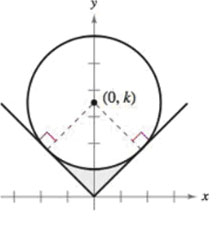Chapter 8.4, Problem 57E

Chapter
Section
Textbook Problem

# Mechanical Design The surface of a machine part is the region between the graphs of y = |x| and x2 + (y – k)2 = 25 (see figure).(a) Find k when the circle is tangent to the graph of y = |x|.(b) Find the area of the surface of the machine part.(c) Find the area of the surface of the machine part as a function of the radius r of the circle.

(a)

To determine

To calculate: The value of k when the circle x2+(yk)2=25 is a tangent to the graph of y=|x|.

Explanation

Given:

The shaded region between the graphs of y=|x| and x2+(yk)2=25 represents the surface of a machine part. Refer the figure given in the question.

Formula used:

The length of diagonal, d, of a square of side ‘a’ is given as,

d=a2+a2=a2

Calculation:

Refer the figure given in the question

(b)

To determine

To calculate: The area of the surface of the machine part.

(c)

To determine

To calculate: The area of the surface of the machine part in terms of radius, r, of the circle.

### Still sussing out bartleby?

Check out a sample textbook solution.

See a sample solution

#### The Solution to Your Study Problems

Bartleby provides explanations to thousands of textbook problems written by our experts, many with advanced degrees!

Get Started

#### What is element if with and

Mathematical Applications for the Management, Life, and Social Sciences

#### The area of the region bounded by , , and r = sec θ is:

Study Guide for Stewart's Single Variable Calculus: Early Transcendentals, 8th

#### True or False: is monotonic.

Study Guide for Stewart's Multivariable Calculus, 8th

#### Define the concept of external validity and a threat to external validity.

Research Methods for the Behavioral Sciences (MindTap Course List)